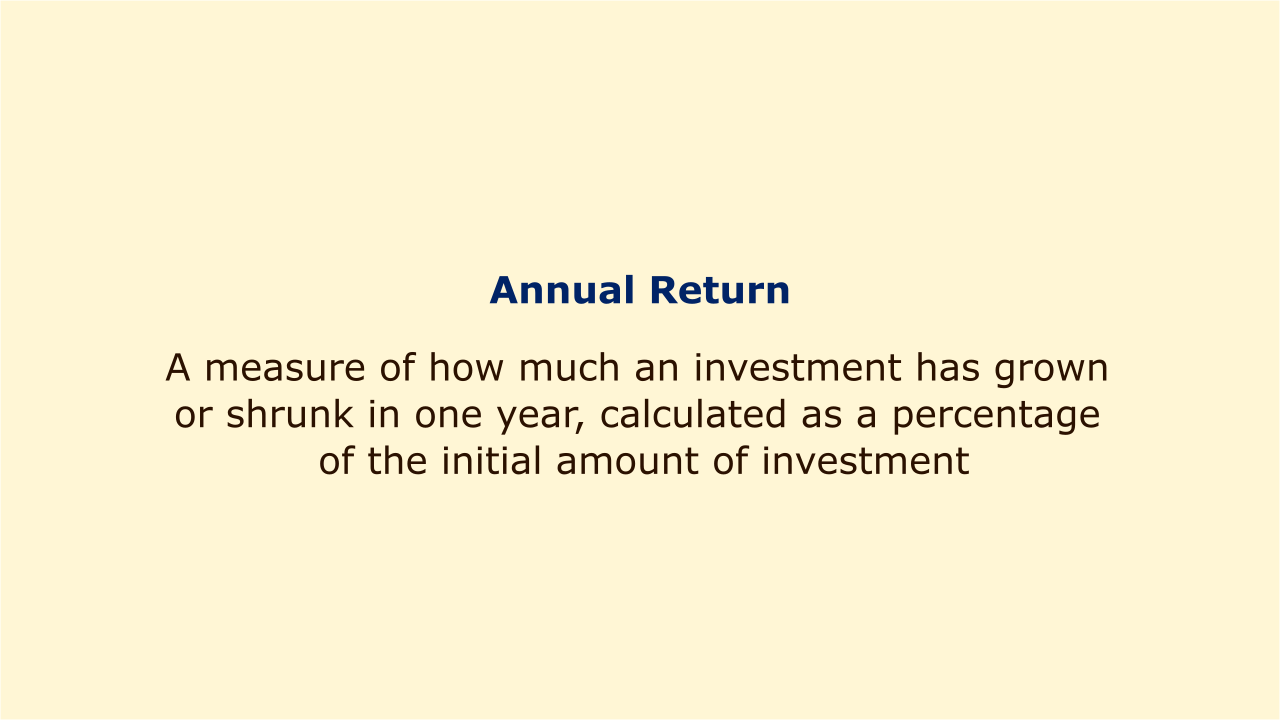# Annual ReturnImage: Moneybestpal.com

### Annual Return measures how much an investment has grown or shrunk in one year, calculated as a percentage of the initial investment.

It may include return sources including dividends, capital returns, and capital appreciation. It is also referred to as a compound annual growth rate or an annualized return (CAGR).

As annual return takes into account compounding and makes allowances for the length of the investment term, it may be used to evaluate the performance of various investments over a range of time periods. It can also be used to assess a fund's or a portfolio's performance over the course of a year.

Knowing the investment's initial and final values, as well as any dividends or interest earned throughout the year, is necessary to compute annual return. The formula for Annual Return is:

Annual Return = ((Final Value - Initial Value + Dividends) / Initial Value) - 1

For illustration, let's say you paid \$10 apiece for 200 shares and after a year, you earned \$1 in cash dividends. By the year's end, the share price was \$9.50. The Annual Return for this investment would be:

Annual Return = ((\$9.50 x 200 + \$1 x 200) - (\$10 x 200)) / (\$10 x 200) - 1

Annual Return = (\$2,100 - \$2,000) / \$2,000 - 1

Annual Return = 0.05 - 1

Annual Return = -0.95 or -95%

This means that the investment lost 95% of its value in one year.

If the investment period is longer or shorter than one year, the Annual Return can be adjusted by using the following formula:

Annualized Return = ((Final Value / Initial Value) ^ (1 / Years)) - 1

Where Years is the holding period in years.

Consider buying 200 shares at \$10 each and holding them for three years as an example. At the conclusion of the third year, the share price was \$12. Each year, you also received \$1 in dividend payments in cash. The Annualized Return for this investment would be:

Final Value = (\$12 x 200 + \$1 x 200 x 3) = \$3,000

Initial Value = (\$10 x 200) = \$2,000

Years = 3

Annualized Return = ((\$3,000 / \$2,000) ^ (1 / 3)) - 1

Annualized Return = (1.5 ^ 0.333) - 1

Annualized Return = 0.144 or 14.4%

This means that the investment grew by an average of 14.4% per year over three years.
Tags